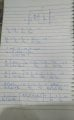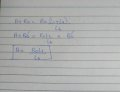# Hartley Oscillator using an Opamp

#### burhanuddin1999

Joined Dec 22, 2020
3
I was trying to derive a model for Opamp Hartley oscillator. I used a R at mouth of opam to increase the output impedance. So i tried including it in calculation. As the opamp is modeled as output voltage of Avi so using a R in series with it with help me to reconfigure the circuit changing the circuit voltage source into a current source using source transformation theorem. Here are my calculations. Can you tell me where i messed up.#### LvW

Joined Jun 13, 2013
1,669
Sorry - but I must state that the opamp-based Hartley oscillator as shown in the linked document does not work. The circuit was derived from a transistor-based oscillator without considering the fact that the opamp is a voltage source (and not a current source). Hence, the inductor L2 acts as a pure load - and has no influence on the feedback path.

#### burhanuddin1999

Joined Dec 22, 2020
3
Sorry - but I must state that the opamp-based Hartley oscillator as shown in the linked document does not work. The circuit was derived from a transistor-based oscillator without considering the fact that the opamp is a voltage source (and not a current source). Hence, the inductor L2 acts as a pure load - and has no influence on the feedback path.
Hi, Yes i am aware of the problem that's why I attached an external resistance, Ro at ouput terminal. Then by using Norton Source transformation i transformed the voltage source Avi into a current source Avi/R

#### LvW

Joined Jun 13, 2013
1,669
Hi, Yes i am aware of the problem that's why I attached an external resistance, Ro at ouput terminal. Then by using Norton Source transformation i transformed the voltage source Avi into a current source Avi/R
And what is your problem now?
I think, it would be helpful to see the circuit diagram your eqivalent small-signal diagram is based on.

#### burhanuddin1999

Joined Dec 22, 2020
3
the problem is A i have calculated is based of Ro and many literature and simulation state otherwise. You can see the calculations above.

#### LvW

Joined Jun 13, 2013
1,669
It really would be helpful to see the circuit diagram.
What is "A"? The loop gain?